# Right circular cone

The volume of a right circular cone is 5 liters. Calculate the volume of the two parts into which the cone is divided by a plane parallel to the base, one-third of the way down from the vertex to the base.

V1 =  0.1852 l
V2 =  4.8148 l

### Step-by-step explanation: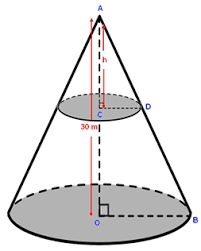Did you find an error or inaccuracy? Feel free to write us. Thank you!

Showing 1 comment:Dr Math
1:3 is the ratio of heights
1:32 = 1:9 is the ratio of the area of base circles... due to two dimensional nature of the area.
1:33 = 1:27 is the ratio of volumes...  .. volume has three-dimensional natureTips to related online calculators
Check out our ratio calculator.
Tip: Our volume units converter will help you with the conversion of volume units.

#### You need to know the following knowledge to solve this word math problem:

We encourage you to watch this tutorial video on this math problem:

## Related math problems and questions:

• ConeCircular cone with height h = 29 dm and base radius r = 3 dm slice plane parallel to the base. Calculate the distance of the cone vertex from this plane, if solids have the same volume.
• Pyramid cutWe cut the regular square pyramid with a parallel plane to the two parts (see figure). The volume of the smaller pyramid is 20% of the volume of the original one. The bottom of the base of the smaller pyramid has a content of 10 cm2. Find the area of the
• ConeCircular cone of height 15 cm and volume 5699 cm3 is at one-third of the height (measured from the bottom) cut by a plane parallel to the base. Calculate the radius and circumference of the circular cut.
• Cube cutIn the ABCDA'B'C'D'cube, it is guided by the edge of the CC' a plane witch dividing the cube into two perpendicular four-sided and triangular prisms, whose volumes are 3:2. Determine in which ratio the edge AB is divided by this plane.
• MO - trianglesOn the AB and AC sides of the triangle ABC lies successive points E and F, on segment EF lie point D. The EF and BC lines are parallel and is true this ratio FD:DE = AE:EB = 2:1. The area of ABC triangle is 27 hectares and line segments EF, AD, and DB seg
• Calculate 3Calculate the cube volume whose edge is 3x-1,3x-1,3x-1
• Cross-sections of a coneCone with base radius 16 cm and height 11 cm divide by parallel planes to base into three bodies. The planes divide the height of the cone into three equal parts. Determine the volume ratio of the maximum and minimum of the resulting body.
• Lateral surface areaThe ratio of the area of the base of the rotary cone to its lateral surface area is 3: 5. Calculate the surface and volume of the cone, if its height v = 4 cm.
• Cutting coneA cone with a base radius of 10 cm and a height of 12 cm is given. At what height above the base should we divide it by a section parallel to the base so that the volumes of the two resulting bodies are the same? Express the result in cm.
• GeodesistTriangle shaped field (triangle ABC) has side AB = 129 m. path XY is parallel to the side AB which divided triangle ABC into two parts with same area. What will be the length of the path XY? Help please geodesist ...
• Axial section of the coneThe axial section of the cone is an isosceles triangle in which the ratio of cone diameter to cone side is 2: 3. Calculate its volume if you know its area is 314 cm square.
• Iglu - cone tentThe cone-shaped tent is 3 m high, the diameter of its base is 3.2 m. a) The tent is made of two layers of material. How many m2 of fabric is needed for production (including flooring), if 20% needs to be added to the minimum amount due to cutting waste? b
• Similarity coefficientThe ratio of similarity of two equilateral triangles is 3.5 (ie 7:2). The length of the side of smaller triangle is 2.4 cm. Calculate the perimeter and area of ​​the larger triangle.
• Cooling liquid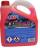Cooling liquid is diluted with water in a ratio of 3:2 (3 parts by volume of coolant with 2 volumes of water). How many coolant volumes must be prepared for a total of 0.7 dm3 (liters) of the mixture?
• MIT 1869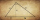You know the length of hypotenuse parts 9 and 16, at which the hypotenuse of a right triangle is divided by a perpendicular running from its opposite vertex. The task is to find the lengths of the sides of the triangle and the length of the line x. This a
• Frustum of a cone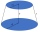A reservoir contains 28.54 m3 of water when full. The diameter of the upper base is 3.5 m, while at the lower base is 2.5 m. Find the height if the reservoir is in the form of a frustum of a right circular cone.
• The base 2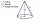The base diameter of a right cone is 16cm and it's slant height is 12cm. A. ) Find the perpendicular height of the cone to 1 decimal place. B. ) Find the volume of the cone, convert to 3 significant figure. Take pi =3.14